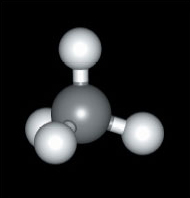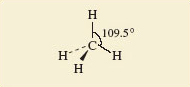Science, Maths & Technology

### Become an OU studentDiscovering chemistry

Start this free course now. Just create an account and sign in. Enrol and complete the course for a free statement of participation or digital badge if available.

# 3 The shapes of some molecules

Let’s now look at the shapes of some simple molecules.

Again consider methane, CH4 (Figure 5)Figure 5 A ball and stick model of methane (CH4)

As an alternative, methane can be represented using so-called 'flying-wedge notation', which is one way of addressing the challenge of representing three-dimensional shapes in two-dimensions either on-screen or on paper.

A flying-wedge representation of the methane molecule is shown in Figure 6.Figure 6 A flying-wedge representation of methane

The atom at the pointed or thin end of the wedge is assumed to be in the plane of the paper, and the atom at the thick end is in front of the plane (this is sticking out towards you). A continuous line (—) joins two atoms that both lie in the plane of the paper. A dashed line (- -) joins together two atoms, one of which is in the plane of the paper, whereas the other is behind it (pointing away from you).

Look back at the ball and stick representation (Figure 5) and compare the two.

You will be seeing the flying wedge approach used to depict the shapes of other molecules in the sections that follow.